×
Get Full Access to UH - CHEM 1332 - Study Guide - Final
Get Full Access to UH - CHEM 1332 - Study Guide - Final

×

UH / Chemistry / CHEM 1332 / What is dipole ­induced dipole?

# What is dipole ­induced dipole? Description

##### Description: This is a review for the chem 2 final exam
17 Pages 70 Views 8 Unlocks
Reviews

Chem Final Review

## What is dipole ­induced dipole ?CHAPTER 10

● SOLUTIONS

○ Homogeneous mixtures ­ consist of SOLUTE and SOLVENT

● ENERGETICS

○ Make solution: BREAK interparticles forces in both solute & solvent, MAKE new interparticle forces. ○ BROKEN ONES CANNOT BE MUCH STRONGER THAN MADE ONES ­ otherwise solution will not form:

○ Always get increase in entropy; can have both exothermic and endothermic.

○ Exo bond making

○ Endo bond breaking

● FORCES

○ Usual ones (ionic, H­bonding, dipole­dipole, LD) when mixing substances that have SAME type of forces

○ When different:

IONIC + H­Bonding or DIPOLE­DIPOLE Ion­dipole Ionic substances + water IONIC + LONDON DISPERSION Ion­induced dipole Rarely happen

## What is the hess’s law?DIPOLE­DIPOLE + London Dipole­induced dipole

ionic ­ nonmetal + metal

Covalent ­ nonmetal + nonmetal

● SOLUTIONS AND EQUILIBRIUM

○ Unsaturated → can add more solute We also discuss several other topics like What is connectome?

○ Saturated → maximum amount of solute ­ usually in contact with undissolved solute ○ Supersaturated → too much solute ­ v. unstable

● QUANTIFYING SOLUTIONS

○ molarity (M) = moles solute / liters of​solution Watch for ionic compounds: ○ molality (m) = moles solute / kg of solvent They DISSOCIATE into ○ mole fraction of X = moles of X / total moles several particles!

○ mass % of X = [mass of X / total mass] x 100

○ density = grams per liters

## What is the ionic redox reactions?● Constants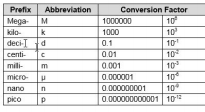○ Energy : 1 CAL = 4.184 J

○ Temp : K = C +273

○ Pressure Units: 1 atm = 760 mmHg = 101.325 kPa =

101325 Pa = 1.01325 bar = 14.7 psi

○ R = 0.0821 L atm / mol K We also discuss several other topics like What was the purpose of sputnik 1?

○ Arrhenius Equation use 8.314 for Ea = kj → Change to J

● C​OLLIGATIVE PROPERTIES

● Depend upon amount of solute (concentration)

● Vapor Pressure DECREASES with increasing concentration ­ Raoult's Law Don't forget about the age old question of What is the musical style of arnold schoenberg?

VP solution = VP solvent x MF solvent

VP solution = (VP solvent x MF solvent) + (VP solute x MF solute)

● Boiling Point INCREASES with increasing concentration

Each solvent has constant (Kbp): 0.512 °C/m for water

CHANGE in boiling point (ΔT​bp​) = K​bp​x molality of solution

(boiling point of solution = boiling point of solvent + change in boiling point)

● Freezing Point DECREASES with increasing concentration

Each solvent has constant (Kfp): 1.86 °C/m for water

CHANGE in freezing point (ΔTf​p​) = K​fp​x molality of solution

(freezing point of solution = freezing point of solvent ­ change in freezing point)

Freezing Point Depression

Freezing point depends on solutes

● Molar Mass of Solute from Point change Molar mass = ​(grams solute x k)

ΔT x kg solvent

ALL OF ABOVE DEPEND UPON NUMBER OF PARTICLES ­ IONIC SPLIT UP INCREASING NUMBER OF PARTICLES SO INCREASE EFFECT We also discuss several other topics like How is the respiratory system organized?
If you want to learn more check out What are homogeneous mixtures?

● Osmotic Pressure ­ Osmosis is flow of solvent from less concentrated solution to more concentrated one. ● Π = molarity x R x T​(R = 0.08206, T = in Kelvin) We also discuss several other topics like An increase in candidates time spent on canvassing in their distress tend to cause an increase in their chances of winning the election. what is the dependent variable in this statement?

● Molar Mass of Solute from Osmotic Pressure Molar mass =​(grams solute x R x T) V x Π

● SOLUBILITY

● Like dissolves Like

○ Water & ethanol (H­Bonds)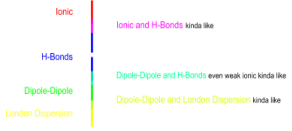○ Heptane & hexane (LD forces)

● Unlike does not dissolve unlike

● Kinda like dissolves kinda like

● Water­ soluble: methanol, ethanol

○ insoluble: heptanol

● Hexane­ soluble: heptanol, ethanol

○ insoluble: methanol

● H­BONDS: N­O­F

● Temperature:

○ As T increases, solubility increases IF ΔH is endothermic

○ As T increases, solubility decreases IF ΔH is exothermic

● Pressure: As P increases, solubility increases (gas only)

○ Henry’s Law: Solubility = K​H ​x Pressure

● Entropy: Small endothermic ΔH: increase in entropy is bigger, so it is soluble

○ Big endothermic ΔH: increase in entropy is smaller, so it is insoluble

■ Exothermic ΔH: increase in entropy is irrelevant, so it is soluble

■ Gases dissolved in liquids or solids​: entropy decreases, always exothermic

CHAPTER 11

Rate of reaction = ​change in concentration​= ​Δ[chemical]

change in time Δt

● Relative Rates

For reaction

a A + b B → c C + d D Rate = ​­​1 Δ[A] ​​=​­​1 Δ[B] ​​= ​1 Δ[C] ​= ​1 Δ[D]

a Δt b Δt c Δt d Δt

● Types of Rate

○ Average rate (over period of time) = (total change in concentration) / (change in time) ○ Instantaneous rate = slope of tangent at a given time to a curve of concentration vs time Initial ○ Rate = instantaneous rate at time = zero

Rate Law

ALWAYS OF FORM rate = k[Reactant A]​x​[Reactant B]​y​…

k = Rate constant temperature dependent

units depend on rest of equation

x, y exponents or order of reaction

Overall order sum of individual orders

Determine rate law by: measuring rate as starting concentration is varied

Plug data into rate law equation → simultaneous equations

divide one by another to eliminate all but one unknown

repeat until all exponents are known

if necessary, plug into one equation to solve for k

● FACTORS THAT AFFECT RATE

○ Phase of reaction and reactants:

■ solid < liquid < solution < gas

○ Subdivision of solid

■ chunks < powder

○ Concentration of reactants

■ As concentration increases, rate increases

○ Temperature

■ As T increases, rate increases

○ Catalyst

■ Speeds up reaction

● Integrated Rate Equations

○ First order ln {[A]​o​­[A]​t​} = kt

○ Second order 1 ​­ ​1 ​= kt

[A]​t​[A]​o

○ Half Life Time for half of reactant to disappear: (1 half life; half gone) (2 half lives; 3/4 gone)

(3 half lives; 7/8 gone)

○ First order t​1/2​= (ln 2) / k​concentration independent

○ Second order t​1/2​= 1 / k[A]​o​concentration dependent

● Arrhenius Equation

○ k = A exp ( ­E​a​/RT) ​or ln K = ln A – E​a​/ RT

○ A = frequency factor (related to probability and orientation of collisions) ○ Ea = activation energy (always endothermic) IN JOULES

○ R = 8.314 J / mol K

○ T = temperature in K

Find Ea and A by plotting ln K against 1 / T or use equation:

​ln( k​1​/ k​2​) = E​a​/R {1/T​2​– 1/ T​1​}​​where 1 and 2 represent k values at different temperatures RATE AND TIME ARE INVERSE!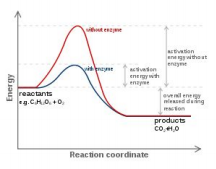● Reaction Coordinate Diagrams

○ Peaks: transition states [activated complexes]

○ Valleys: intermediate, highest peak slowest step

○ Ea: energy between reactants and highest peak

● Mechanisms

○ Reactions occur in step(s) mechanism(s)

■ each step is balanced chemical equation

■ each step should have one or two reactants [more is unlikely collision]

■ all steps must add up to the overall equation

■ one step is slowest: RATE DETERMINING STEP

■ during mechanism: INTERMEDIATES are made then used

■ CATALYSTS ​are used then made

■ Lower Ea

○ rate law comes directly from rate determining step

■ reactants in RDS are those used in rate law

■ coefficients of reactants in RDS are exponents in rate law

■ if have intermediate in RDS, replace by reactants in previous steps that made it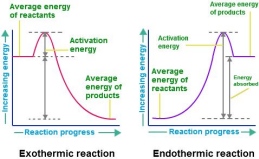CHAPTER 12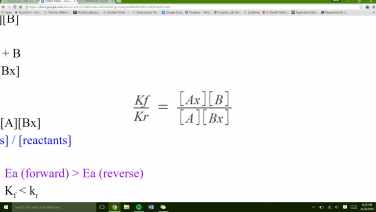● Forward reaction:

○ Ax + B → A + Bx

○ ratefor = kf[Ax][B]

● Reverse reaction:

○ A + Bx → Ax + B

○ raterev = kr[A][Bx]

● At equilibrium:

○ ratefor = raterev

○ kf[Ax][B] = kr[A][Bx]

○ Keq = [products] / [reactants]

● Endothermic Ea (forward) > Ea (reverse)

Kf < kr

Keq< 1

More reactants than products

● Exothermic Ea (forward) < Ea (reverse)

Kf > kr

Keq< 1

More products than reactants

*Equilibrium is Thermodynamic*

● aA + bB → cC + dD

● Reaction Line

○ aA + bB ←→ cC + dD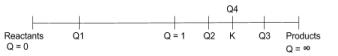Q = 1

Q < K

Reaction will go to the right

Forward reaction faster

Q1

Q < K

Reaction will go to the right

Forward reaction faster

Q2

Q < K

Reaction will go to the right

Forward reaction faster

Q3

Q > K

Reaction will go to the left

Reverse reaction faster

Q4

Q = K

Reaction is at equilibrium

Both reactions at same rate

● Properties of K

○ Flip equation k → 1/k

○ Multiply by a factor of x k → kx

○ Add equations Multiply k values

● Phases and K

○ Do not use solids or pure liquids in equations

○ Gases: aA + bB ←→ cC + dD

● Kp and Kc

○ Kp = (Kc)(RT)Δn

● SCE Charts

○ Start, Change, Equilibrium● Le Chatelier’s Principle

■ Q < K

■ Reaction goes to the right

■ Use reactants, make products

■ Q > K

■ Reaction goes to the left

■ Use products, make reactants

○ Remove reactant

■ Q > K

■ Reaction goes to the left

■ Make reactants, use products

○ Remove product

■ Q < K

■ Reaction goes to the right

■ Make products, use reactants

IF A REACTION AT EQUILIBRIUM IS DISTURBED, THE REACTION WILL MOVE IN SUCH A WAY AS TO RESTORE THE EQUILIBRIUM

● Temperature

○ Heat a reaction

■ Reaction wants to remove the heat

■ Use heat

■ Go in endothermic direction

■ Add heat → Add pressure → reaction goes to left → endothermic

○ Cool a reaction

■ Reaction wants to replace the heat

■ Make heat

■ Go in exothermic reaction

■ Remove heat → remove pressure → reaction goes to right → exothermic

○ ΔH negative → ln(k) ∝ 1/k → Temperature increases, K decreases → goes to R ○ ΔH positive → ln(k) ∝ ­1/k → Temperature increases, K increases → goes to P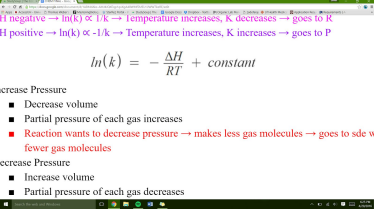● Pressure

○ Increase Pressure

■ Decrease volume

■ Partial pressure of each gas increases

■ Reaction wants to decrease pressure → makes less gas molecules → goes to sde with fewer gas molecules

○ Decrease Pressure

■ Increase volume

■ Partial pressure of each gas decreases

■ Reaction wants to increase pressure → makes more gas molecules → goes to sde with more gas molecules

● Catalysts

○ Speed up reaction

○ No effect on equilibrium

● Addition of inert gas○ Changes overall pressure

○ No change on partial pressure

○ No effect on equilibrium

CHAPTER 13

● Acids

○ Produce H+in water

○ Two Types:

■ Binary: monatomic anion + H+

Name: take anion name, add “hydro” as prefix, change “ide” to

“ic acid”

Ex) HCl → chloride → hydrochloric acid

■ Oxy/Ternary: Oxyanion + H+

Name: take anion name, change “ate” to “ic acid”, change “ite”

to “ous acid”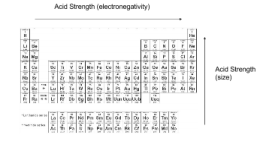Ex) HClO3 → chlorate → chloric acid

Ex) HClO → hypochlorite → hypochlorous acid

Examples: CN­: cyanide → HCN: hydrocyanic acid

N3­: azide → hydrazoic acid

○ Organic Acids: HCOO­: formate → HCOOH: formic acid CH3COO­: acetate → CH3COOH: acetic acid

○ Carboxylic Acids: ­ane goes to ­anoic acid

Ex) Methane → methanoic acid

Ex) Ethane → ethanoic acid

○ Cations are acids: Conjugate acid of B

Metal cations

○ Strong Acids: (completely dissociate)

■ HCl(aq) → hydrochloric acid

■ HBr(aq)→ hydrobromic acid

■ HI(aq)→ hydroiodic acid

■ HNO3(aq) → nitric acid

■ HClO4(aq) → perchloric acid

■ H2SO4(aq) → sulfuric acid

○ Weak Acids: (do not completely dissociate)

■ HF(aq)→ hydrofluoric acid

■ HCO2H(aq) → formic acid

■ CH3CO2H(aq) → acetic acid

■ H3PO4(aq) → phosphoric acid

■ HClO3(aq) → chloric acid

■ HSO4­(aq) → bisulfate

○ Strong Acids: ka >> 1

■ HA(aq) → H+(aq) + A­(aq)

○ Weak Acids: kais small

■ HA(aq) ←→ H+(aq) + A­(aq)

○ Percent ionization

■ Assume 100% for strong acid

■ For weak acid, percent ionization increases as [acid] decreases

○ pH

■ pH = ­log([H+]eq)

○ Bronsted­Lowry Acids

■ HA(aq) + H2O(l) ←→ H3O+(aq) + A­(aq)

■ Acids are proton (H+) donors

● HA(aq) + B ←→ BH+(aq) + A­(aq)

○ Polyprotic Acids

■ HnX(aq) + H2O(l) ←→ H3O+(aq) + (Hn­1X)­(aq)

■ (Hn­1X)­(aq) + H2O(l) ←→ H3O+(aq) + (Hn­2X)2­(aq) ■ (Hn­2X)2­(aq) + H2O(l) ←→ H3O+(aq) + (Hn­3X)3­(aq)

■ K​a1​(H​n​X) >> K​a2​(H​n​X) >> K​a3​(H​n​X)

● Bases

○ Produce OH­in water

○ Strong Bases: (completely dissociate in water) ■ LiOH(aq) → Lithium hydroxide

■ NaOH(aq) → Sodium hydroxide

■ KOH(aq) → Potassium hydroxide

■ Ba(OH)2(aq) → Barium hydroxide

■ Sr(OH)2(aq) → Strontium hydroxide

○ Weak Acids: (do not completely dissociate)

■ NH3 → Ammonia

■ Metal hydroxides

■ Amines

■ Anions

○ Arrhenius

■ MOH(aq) ←→ M+(aq) + OH­(aq)

○ pOH = ­log([OH­](eq))

○ Strong bases: kb >> 1

○ Weak bases: kbis small

○ Bronsted­Lowry Bases

■ B(aq) + H2O(l) ←→ OH­(aq) + BH+(aq)

■ Bases are proton (H+) acceptors

● HA(aq) + B ←→ BH+(aq) + A­(aq)

● Acid Base Reactions

○ Strength of acid is inversely proportional to strength of conjugate base

○ Water is amphiprotic (acts as both acid and base)

● Neutralization○ Acid + base → water + salt

○ Net ionic equation:

■ H+ + OH­ → H2O

● Buffers and Titrations

○ Correct concentrations of starting solutions

■ M1V1 = M2V2

■ M = n/L

■ New [X] = ((Molarity) x (Volume of X)) / (total Volume)

○ Remove spectator ions

■ Li+, Na+, K+,Rb+, Cs+, Sr+, Ba+

■ Cl­, Br­, I­, NO3­, ClO4­

○ Neutralize

■ Acid + base ←→ salt + water

○ Identify and calculate

■ SCAN chart (start, change, after neutralization)

● Buffers

○ 1:10 or 10:1 acid:base ratio

○ Ideal buffer: 1:1

● Titrations

○ Strong Acid/Strong Base○ Weak Acid/Strong Base○ Weak Base/Strong AcidChapter 15­ Solubility

● Q < Ksp Reaction goes to the right

Can dissolve more solid

Unsaturated

● Q = Ksp Reaction is at equilibrium

Cannot dissolve more solid

Saturated

● Q > Ksp Reaction goes to left

Precipitate in contact with saturated solution

Chapter 16­ Thermodynamics

● First Law of Thermodynamics: ΔH

○ Heat of Reaction

■ Exothermic reactions:

● Give off heat and warm up the surroundings

● Ex) Combustion reactions

● ­ΔH

● Heat of vaporization

■ Endothermic reactions:

● Give off “cold” and cool down the surroundings, absorbs heat.

● Ex) cold packs

● +ΔH

● Heat of fusion

○ Heat of formation

■ Standard State Pure solid or liquid

1M solutions

Gases at 1 atm

Usually 25°C

■ ΔH°f(x) kj/mol

■ H2,N2, O2, F2, Cl2 → gas

■ Br2 → liquid

■ I2, P4, As4, S8, C (graphite) → solid

■ Metals are solid except Hg(l), [Cs and Ga], noble gases

○ Hess’s Law

■ State function

● Change independent of path

● Reversible change

● A + B → C ΔH1

● C → D ΔH2

● A + B → D ΔH1 + ΔH2

● A + B → C + D ΔH1

● C + D → A + B ­ΔH1

● Flip equation → change sign

● Multiply equation, multiply ΔH

■ ΔH reaction:

● ∑{ΔH°f(products)} ­ ∑{ΔH°f(reactants)}

● ΔH°f(element) = 0

● Second Law of Thermodynamics: ΔS to ΔG

○ Spontaneous reactions take place without continual input of energy ○ Entropy of Universe, Suniv ΔSuniv > 0 → spontaneous

ΔSuniv < 0 → nonspontaneous

ΔSuniverse = ΔSsystem + ΔSsurroundings

ΔSsurr∝ ΔHrxn

ΔG​rxn ​= ΔH​rxn​+ TΔS​rxn

ΔGrxn < 0 → spontaneous

ΔGrxn > 0 → nonspontaneous

○ ΔG (kj)

■ Free energy of reaction + endergonic → nonspontaneous ­ exergonic → spontaneous

■ ∑{ΔG°f(products)} ­ ∑{ΔG°f(reactants)}

■ ΔG°f(element) = 0

○ Temperature of Spontaneity change: Set ΔG = 0

T = ΔH°/ΔS°

At equilibrium, ΔG = 0

○ Phase change entropy

■ Boiling point: Before → nonspontaneous

After → Spontaneous

At BP → ΔG = 0

BP = ΔH°vap/ΔS°vap

● Third Law of Thermodynamics: Entropy ΔS

○ S of a substance cannot be zero, except on a perfect crystal at 0 K. ○ Units: J/mol k

○ Phase: S < L < G

○ Pure vs. Mixture: Pure < Mixture

○ Temperature: Increase T, increase S

○ Pressure: Increase P, decrease S

○ Molecule Size: Increase size, increase S

○ Positive if S increases

○ Negative if S decreases

○ ΔS = ∑{ΔS°(products)} ­ ∑{ΔS°(reactants)}

○ ΔS (element) ≠ 0

● Equilibrium and ΔG Reaction line

ΔG ∝ ln(Q/K)

ΔG = RTln(Q/K)

ΔG = RTln(Q) ­ RTln(K)

At Standard state:

Q = 1

ΔG° = ­RTln(K)

Chapter 17­ Redox

● Oxidation and Reduction

○ Oxidation Gain oxygen

Lose hydrogen

Lose electrons

○ Reduction Lose oxygen

Gain hydrogen

Gain electrons

● Ionic redox reactions

○ Identify what is being oxidized and reduced

○ Balance equation → must have equal numbers of electrons lost and gained

● Non­ionic redox reactions

○ Assign oxidation numbers (fake charges)

■ Metal cations → charge on cations Alkali = +1

Alkaline earth = +2

Al, Ga = +3

■ Non­metals F = ­1

H = +1

O = ­2

■ If you still cannot assign oxidation numbers, assign appropriate negative charge to unassigned atom closest to F.

○ Identify what is being oxidized and reduced

○ Balance electron transfer

■ Balance redox atoms using appropriate coefficients on whole species

● Reducing agent​­ Loses electrons and is oxidized in the reaction.

● Oxidizing agent­ ​Gains electrons and is reduced in the reaction.

● Half­equations

○ Eliminate spectator ions

○ Identify what is being oxidized and reduced

○ Balance electrons

○ In acidic/basic solutions Assign oxidation numbers

Identify if being oxidized or reduced

Balance charges with H+(acidic) or OH­(basic)

Balance number of oxygen atoms

Check number of hydrogen atoms

● Potential

○ Positive potential is good

○ +E°red → better oxidizing agent

○ +E°ox → better reducing agent

● Cell potential (V)

○ E°cell = E°red + E°ox

● Galvanic Cells

○ Ex) Zn(s)+Cu2+(aq)→Zn2+(aq)+Cu(s)

○ Zn(s)|Zn2+(aq)||Cu2+(aq)|Cu(s)

● E and ΔG

○ Potential

■ ΔG° = ­nFE°

● F (Faraday) = 96500 J/mol e­ V

■ E° = (RT/nF)lnk

● Electrolytes

○ Metal → cation + electron(s)

■ Oxidation

○ Nonmetal + electron(s) → anion

■ Reduction

○ Metal + nonmetal → ionic compound

■ Spontaneous redox equation

○ Cation + electron(s) → metal

■ Reduction

○ Anion → nonmetal + electron(s)

■ Oxidation

○ Ionic compound → metal + nonmetal

■ Nonspontaneous redox equation

■ Need continual input of energy

● Electrolytic cell

● Molten ionic compounds

○ Cathode: cation → element

○ Anode: anion → element

● Aqueous ionic compounds

○ Cathode:

■ Cation → element

■ 2H2O + 2e­ → H2 + 2OH­(aq)

○ Anode:

■ Anion → element

■ 2H2O → O2 + 4e­ + 4H+(aq)

● Equations:

○ Charge (C) = current (amps) x time (sec) ○ 1 mol e­ = 96500 C = 1 Faraday

Page ExpiredIt looks like your free minutes have expired! Lucky for you we have all the content you need, just sign up here